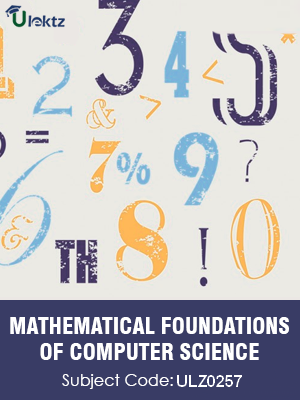•My WalletMy Order
•My Profile
•My Connections
•My Books
•My Videos
•My Tests
•My Calender
•My Messages
•My Shopping Cart
•My Orders
•Account Settings
•Help

# Book Details# Mathematical Foundations of Computer Science

 Course Code : ULZ0257 Author : uLektz University : General for All University Regulation : 2017 Categories : Computer Science Format :ePUB3 (DRM Protected) Type : eBook

FREE

Description :Mathematical Foundations of Computer Science of ULZ0257 covers the latest syllabus prescribed by General for All University for regulation 2017. Author: uLektz, Published by uLektz Learning Solutions Private Limited.

Note : No printed book. Only ebook. Access eBook using uLektz apps for Android, iOS and Windows Desktop PC.

##### Topics
###### Unit I: Mathematical Logic :

1.1 Propositional Calculus: Statements and Notations - Connectives - Truth Tables

1.2 Tautologies - Equivalence of Formulas, Duality law - Tautological Implications

1.3 Normal Forms - Theory of Inference for Statement Calculus

1.4 Consistency of Premises - Indirect Method of Proof

1.5 Predicate calculus: Predicative Logic

1.6 Statement Functions, Variables and Quantifiers - Free & Bound Variables - Inference theory for predicate calculus

###### Unit II : Number Theory & Induction:

2.1 Properties of integers - Division Theorem - The Greatest Common Divisor

2.2 Euclidean Algorithm - Least Common Multiple - Testing for Prime Numbers

2.3 The Fundamental Theorem of Arithmetic - Modular Arithmetic (Fermat’s Theorem and Euler ‘s Theorem) - Mathematical Induction: Principle of Mathematical Induction, exercises

###### Unit III: Set Theory:

3.1 Introduction, Operations on Binary Sets

3.2 Principle of Inclusion and Exclusion

3.3 Relations: Properties of Binary Relations - Relation Matrix and Digraph - Operations on Relations, Partition and Covering

3.4 Transitive Closure, Equivalence, Compatibility - Equivalence - Compatibility

3.5 Partial Ordering Relations - Hasse Diagrams

3.6 Functions: Bijective Functions - Composition of Functions

3.7 Inverse Functions, Permutation Functions - Permutation Functions

3.8 Recursive Functions

###### Unit IV: Graph Theory:

4.1 Basic Concepts of Graphs

4.2 Sub graphs

4.3 Matrix Representation of Graphs: Adjacency Matrices - Incidence Matrices

4.4 Isomorphic Graphs

4.5 Paths and Circuits - Eulerian and Hamiltonian Graphs

4.6 Multigraphs, (Problems and Theorems without proofs) - Planar Graphs

4.7 Euler’s Formula

4.8 Graph Colouring and Covering

4.9 Chromatic Number ( Problems and Theorems without proofs)

4.10 Trees - Directed trees - Binary Trees - Decision Trees

4.11 Spanning Trees: Properties - Algorithms for Spanning trees and Minimum Spanning Tree

###### Unit V: Algebraic Structures:

5.1 Algebraic Structures: Lattice: Properties - Lattices as Algebraic Systems

5.2 Algebraic Systems with one Binary Operation - Properties of Binary operations

5.3 Semi groups and Monoids - Homomorphism of Semi groups and Monoids

5.4 Groups: Abelian Group - Cosets, Subgroups (Definitions and Examples of all Structures) - Algebraic Systems with two Binary Operations: Rings

5.5 Combinatorics: Basic of Counting - Permutations, Combinations

5.6 Derangements - Permutations with Repetition of Objects - Circular Permutations - Restricted Permutations - Restricted Combinations - Pigeonhole Principle and its Application

5.7 Binomial Theorem: Binomial and Multinomial Coefficients

5.8 Generating Functions of Permutations and Combinations

5.9 The Principles of Inclusion – Exclusion

###### Unit VI: Recurrence Relation:

6.2 Partial Fractions - Calculating Coefficient of Generating Functions Recurrence Relations

6.3 Formulation as Recurrence Relations - Solving linear homogeneous recurrence Relations by substitution

6.4 Generating functions and The Method of Characteristic Roots - Solving Inhomogeneous Recurrence Relations